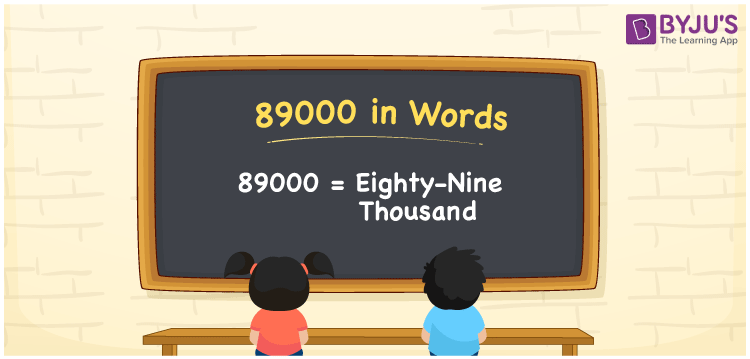# 89000 in Words

The number 89000 stands for Eighty-nine Thousand. The place value table is extremely useful when converting numbers to words. As a result, you may conclude that writing 89000 in words using the place value table is preferable. For example, if you did a payment of Rs. 89000, you may write “I did a payment of Rs. Eighty-nine Thousand”. Let us just look at how to write 89000 in words, as well as the procedures that must be performed, in detail.

 89000 in Words: Eighty-nine Thousand. Eighty-nine Thousand in Numerical Form: 89000.

## 89000 in English Words## How to Write 89000 in Words?

Use a place value chart to convert 89000 to words. The number 89000 has a place value table as well as an expanded form, which is provided below.

 Ten-thousands Thousands Hundreds Tens Ones 8 9 0 0 0

The number 89000 is written as follows, using the place value chart:

= 8 × Ten thousand + 9 × Thousand + 0 × Hundred + 0 × Ten + 0 × One

= 8 × 10000 + 9 × 1000

= 80000 + 9000

= 89000

= Eighty-nine thousand

Hence, 89000 in words is Eighty-nine thousand.

The natural number 89000 exists after 88999 but before 89001.

89000 in words – Eighty-nine thousand

Is 89000 an odd number? – No

Is 89000 an even number? – Yes

Is 89000 a perfect square number? – No

Is 89000 a perfect cube number? – No

Is 89000 a prime number? – No

Is 89000 a composite number? – Yes

## Frequently Asked Questions on 89000 in Words

Q1

### Write 89000 in words.

89000 in words is eighty-nine thousand.

Q2

### Simplify 85000 + 4000, and express in words.

Simplifying 85000 + 4000, we get 89000. Hence, 89000 in words is eighty-nine thousand.

Q3

### Convert eighty-nine thousand into numbers.

Eighty-nine thousand in numbers is 89000.